# Division by zero facts for kids

Kids Encyclopedia Facts

In mathematics, a number can not be divided by zero. Observe:

1.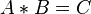$A * B = C$

If B = 0, then C = 0. This is true. But:

2.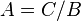$A = C/B$

(where B=0, so we just divided by zero)

Which is the same as:

3.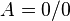$A = 0/0$

The problem is that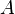$A$ could be any number. It would work if$A$ were 1 or if it were 1,000,000,000. 0/0 is said to be of "indeterminate form" for this reason, because it has no single value. Numbers of the form A/0, on the other hand, where$A$ is not 0, are said to be "undefined", or "undeterminated." This is because any attempt to define them will result in a value of infinity, which is itself undefined. Usually when two numbers are equal to the same thing, they are equal to each other. That is not true when the thing they are both equal to is 0/0. This means that the normal rules of maths do not work when the number is divided by zero.

## Incorrect proofs based on division by zero

It is possible to disguise a special case of division by zero in an algebraic argument. This can lead to invalid proofs, such as 1=2, as in the following:

With the following assumptions: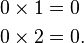\begin{align} 0\times 1 &= 0 \\ 0\times 2 &= 0. \end{align}

The following must be true: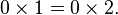$0\times 1 = 0\times 2.\,$

Dividing by zero gives: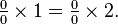$\textstyle \frac{0}{0}\times 1 = \frac{0}{0}\times 2.$

Simplify: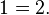$1 = 2.\,$

The fallacy is the assumption that dividing by 0 is a legitimate operation with 0/0 = 1.

Most people would probably recognize the above "proof" as incorrect, but the same argument can be presented in a way that makes it harder to spot the error. For example, if 1 is written as x, then 0 can be hidden behind x-x and 2 behind x+x. The above-mentioned proof can then be displayed as follows: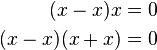\begin{align} (x-x)x = 0 \\ (x-x)(x+x) = 0 \end{align}

therefore: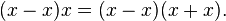$(x-x)x = (x-x)(x+x).\,$

Dividing by x - x gives: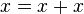$x = x+x\,$

and dividing by x gives:$1 = 2.\,$

The "proof" above is incorrect because it divides by zero when it divides by x-x, because any number minus itself is zero.

## Calculus

In calculus, the above "indeterminate forms" also come as a result of direct substitution while evaluating limits.

## Division by zero in computers

If a computer program tries to divide an integer by zero, the operating system will usually detect this and stop the program. Usually it will print an "error message," or give the programmer advice on how to improve the program. Division by zero is a common bug in computer programming. Dividing floating point numbers (decimals) by zero will usually result in either infinity or a special NaN (not a number) value, depending on what is being divided by zero.

## Division by zero in geometry

In geometry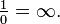$\textstyle \frac{1}{0} = \infty.$ This infinity (projective infinity) is neither a positive or a negative number, the same way that zero is neither a positive or negative numberDivision by zero Facts for Kids. Kiddle Encyclopedia.